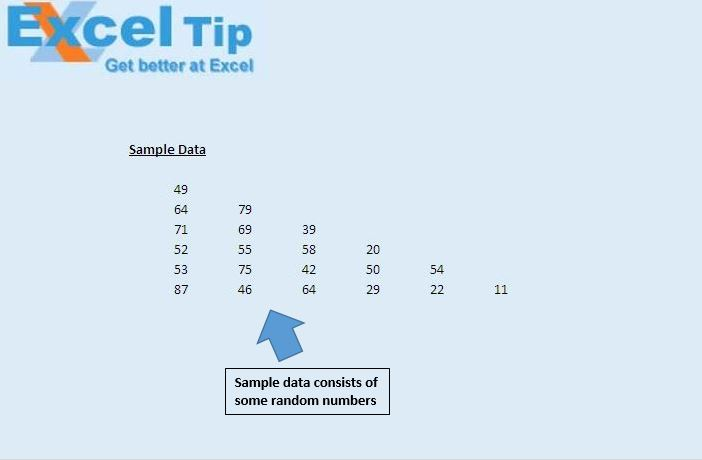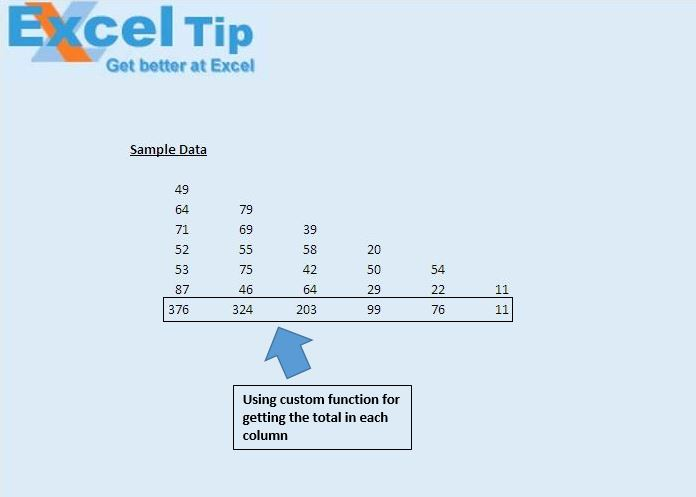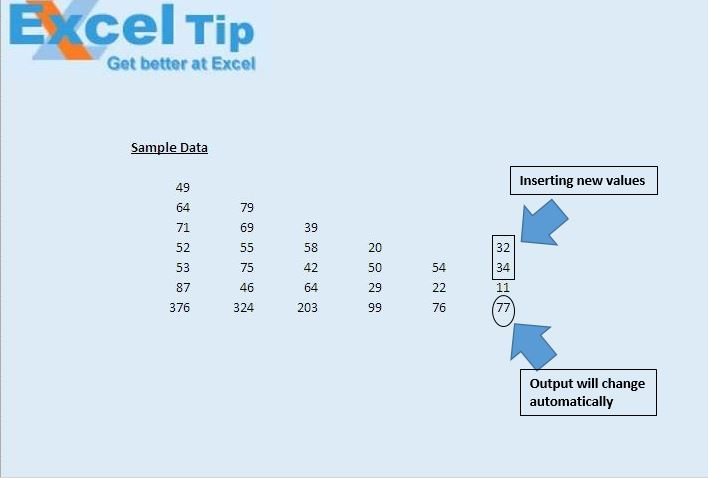# Generate total for dynamic area using VBA in Microsoft Excel

In this article, we will create a custom function to generate the total for the dynamic range.In this example, we want to calculate the sum of values in all the cells in each column. Each column has different number of values.

We have created a custom function ‘DynaSum’ to calculate the sum of values in all the cells.Custom function ‘DynaSum’ calculates the sum of values of all the consecutive cells. This function will sum all the values until blank cell is encountered.Code explanation

Set Rng = Application.Caller

Above code is used to assign cell containing the custom function as Range object.

```
Option Explicit

Function DynaSum(Optional Rng As Range)

'Declaring variables
Dim Total As Double
Dim LngRow As Long
Dim LngCol As Integer

'Refresh function when value in worksheet is changed
Application.Volatile

'Assigning range if Range is not specified in the function
If Rng Is Nothing Then
Set Rng = Application.Caller
End If

'Getting the column and row number
LngCol = Rng.Column
LngRow = Rng.Row - 1

'Looping through all the cells in the column until blank cell is encountered
Do Until IsEmpty(Cells(LngRow, LngCol))
Total = Total + Cells(LngRow, LngCol)
LngRow = LngRow - 1
If LngRow = 1 Then Exit Do
Loop

'Getting output
DynaSum = Total

End Function

```